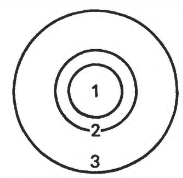# Probability of firing exactly one shot in each annular zone

• B
• WMDhamnekar
In summary, the probability of exactly one shot landing in each zone of a circular target with radii in the ratio of 1:1/2:1/3 is equal to 5/432. However, the author's answer of 5/72 accounts for all possible permutations, assuming no misses.f

#### WMDhamnekar

MHB
TL;DR Summary
Suppose a circular target is divided into three zones bounded by concentric circles of radius 1/3, 1/2, and 1, as illustrated in the following diagram.
If three shots are fired at random at the target, what is the probability that exactly one shot lands in each zone?My attempt to answer this question: With the radii in the ratio ## 1: \frac12: \frac13 ##, the area of the corresponding circles will be in the ratio of ##1: \frac14: \frac19 ##. The areas of the three rings will be in the ratio of ## \frac34 : \frac{5}{36}: \frac19 ##
So, if three shots are fired at random at the target, the probability that exactly one shots lands in each zone is equal to ## \frac34 \times \frac{5}{36} \times \frac19 = \frac{5}{432}## But author said the answer is ##\frac{5}{432}\times 3! = \frac{5}{72}## How is that?

Would any member of physics forum provide me a satisfactory explanation?

Summary: Suppose a circular target is divided into three zones bounded by concentric circles of radius 1/3, 1/2, and 1, as illustrated in the following diagram.
If three shots are fired at random at the target, what is the probability that exactly one shot lands in each zone?

View attachment 314939
My attempt to answer this question: With the radii in the ratio ## 1: \frac12: \frac13 ##, the area of the corresponding circles will be in the ratio of ##1: \frac14: \frac19 ##. The areas of the three rings will be in the ratio of ## \frac34 : \frac{5}{36}: \frac19 ##
So, if three shots are fired at random at the target, the probability that exactly one shots lands in each zone is equal to ## \frac34 \times \frac{5}{36} \times \frac19 = \frac{5}{432}## But author said the answer is ##\frac{5}{432}\times 3! = \frac{5}{72}## How is that?

Would any member of physics forum provide me a satisfactory explanation?
You've calculated the probability that the first shot lands in the outer area, the second lands in the middle area and the third lands in the inner area. That is not the only way you can have one in each area.

••Dale and WMDhamnekar
You've calculated the probability that the first shot lands in the outer area, the second lands in the middle area and the third lands in the inner area. That is not the only way you can have one in each area.
Using above comment, there are 6 possible permutations, giving the correct answer. Assuming no misses.

•PeroK